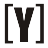# kilovolt-amps (kVA) to Amps (A) Calculator

To calculate kVA to Amps, select phase (single or three phase) first, insert value of kilovolt-amps and volts then press Calculate Button to get the result of Amps (A). Swap to Amps to kVA calculator.

 Select phase #: Single phase Three phase Enter kilovolt-amps: kVA Enter volts: V Result in amps: A

## How to Calculate kilovolt-amps (kVA) to Amps (A)

Single phase kVA to A Formula
`I (A) = 1000 × S (kVA) / V (V)`

I in amps equal to 1000 multiply the apparent power S in kVA, divided by the voltage V in volts.

Three phase kVA to A Formula
Line to line voltage

`I (A) = 1000 × S (kVA) / (√3 × VL-L (V) )`

I in amps (with balanced loads) equal to 1000 multiply the apparent power S in kVA, divided by the square root of 3 times the line to line RMS voltage VL-L in volts.

Line to neutral voltage

`I (A) = 1000 × S (kVA) / (3 × VL-N (V) )`

I in amps (with balanced loads) equal to 1000 multiply the apparent power S in kVA, divided by 3 times the line to neutral RMS voltage VL-N in volts.YoosFuhl.com Would you like to receive notifications on latest updates? No Yes